Prev Up Next

# Double pairs

Given two pairs between the same two squares, one concludes that these two squares only contain the two digits involved and no other digit. Thus,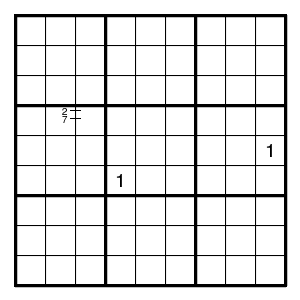implies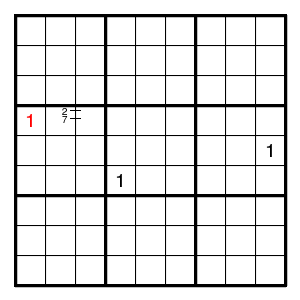Knowledge about what digits can occur in a given square can also be written down explicitly, as in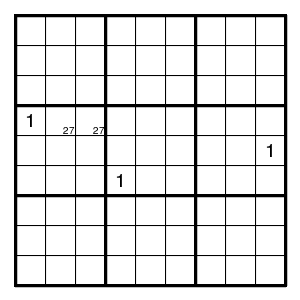For example, in the situation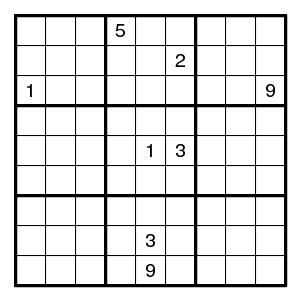one concludes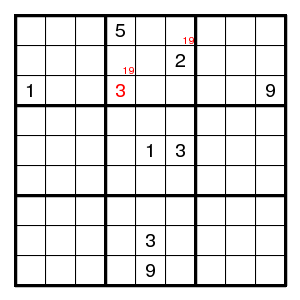Similar things apply in the situation where there are three (or four) digits that can only be on three (or four) positions in a given row, column, or box: these positions must contain these digits and nothing else. See the section on Matching. For an example where this argument (with three digits) is needed, see below.